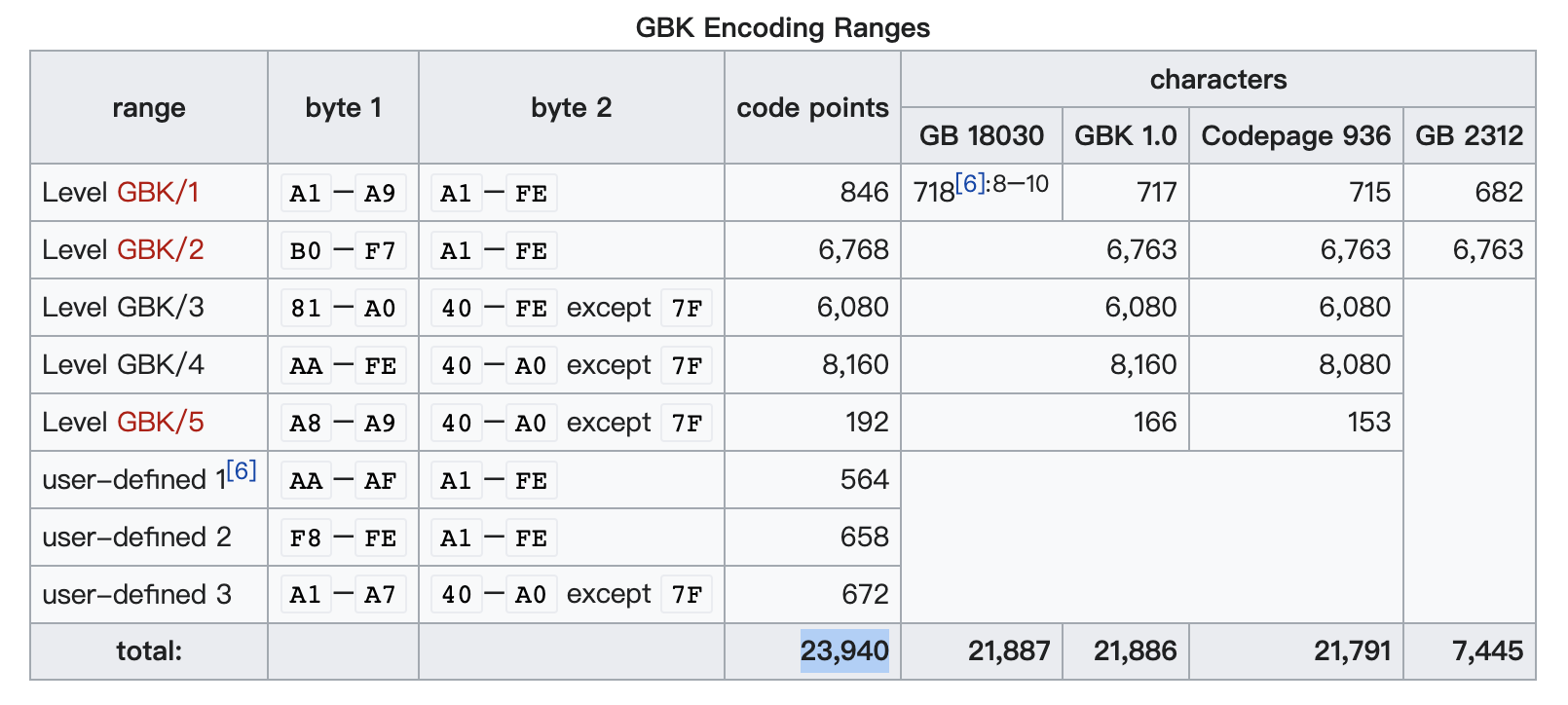JS 字符串转 GBK 编码超精简实现 - EtherDream

2022-8-3 16:22:0 Author: www.cnblogs.com(查看原文) 阅读量:9 收藏

## 前言

JS 中 GBK 编码转字符串是非常简单的，直接调用 `TextDecoder` 即可：

``````const gbkBuf = new Uint8Array([196, 227,   186, 195,   49, 50, 51])
new TextDecoder('gbk').decode(gbkBuf)   // "你好123"
``````

## 遍历https://en.wikipedia.org/wiki/GBK_(character_encoding)#Encoding

``````const ranges = [
[0xA1, 0xA9,  0xA1, 0xFE],
[0xB0, 0xF7,  0xA1, 0xFE],
[0x81, 0xA0,  0x40, 0xFE],
[0xAA, 0xFE,  0x40, 0xA0],
[0xA8, 0xA9,  0x40, 0xA0],
[0xAA, 0xAF,  0xA1, 0xFE],
[0xF8, 0xFE,  0xA1, 0xFE],
[0xA1, 0xA7,  0x40, 0xA0],
]
const codes = new Uint16Array(23940)
let i = 0

for (const [b1Begin, b1End, b2Begin, b2End] of ranges) {
for (let b2 = b2Begin; b2 <= b2End; b2++) {
if (b2 !== 0x7F) {
for (let b1 = b1Begin; b1 <= b1End; b1++) {
codes[i++] = b2 << 8 | b1
}
}
}
}
const str = new TextDecoder('gbk').decode(codes)

// 编码表
const table = new Uint16Array(65536)
for (let i = 0; i < str.length; i++) {
table[str.charCodeAt(i)] = codes[i]
}
``````

## 查表

``````function stringToGbk(str) {
const buf = new Uint16Array(str.length)
for (let i = 0; i < str.length; i++) {
const code = str.charCodeAt(i)
buf[i] = table[code]
}
return new Uint8Array(buf.buffer)
}

stringToGbk('你好')   // [196, 227,   186, 195]
``````

``````function stringToGbk(str) {
const buf = new Uint8Array(str.length * 2)
let n = 0

for (let i = 0; i < str.length; i++) {
const code = str.charCodeAt(i)
if (code < 0x80) {
buf[n++] = code
} else {
const gbk = table[code]
buf[n++] = gbk & 0xFF
buf[n++] = gbk >> 8
}
}
return buf.subarray(0, n)
}

stringToGbk('你好123')    // [196, 227,   186, 195,   49, 50, 51]
``````

## 完善

``````const gbkBuf = new Uint8Array([0x80])
new TextDecoder('gbk').decode(gbkBuf)   // "€"
``````